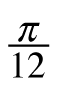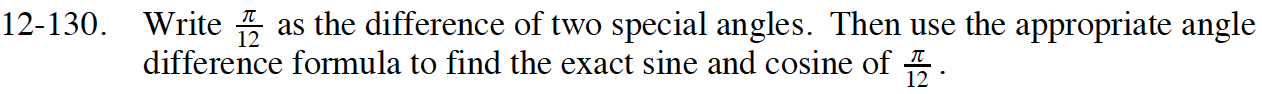Home > CCA2 > Chapter 12 > Lesson 12.2.3 > Problem12-130

12-130.

Writeas the difference of two special angles. Then use the appropriate angle difference formula to find the exact sine and cosine of. Homework Help ✎$\text{Since }\frac{\pi}{3}-\frac{\pi}{4}=\frac{4\pi}{12}-\frac{3\pi}{12}=\frac{\pi}{12},$

you can use the Angle Difference Identity.
Refer to the Math Notes in Lesson 12.2.3.

Substitute the angles into the Angle Difference Identity.

$\text{sin }\left (\frac{\pi}{3}-\frac{\pi}{4} \right )=\text{ sin }\left (\frac{\pi}{3} \right ) \cdot \text{cos } \left (\frac{\pi}{4} \right ) - \text{cos} \left (\frac{\pi}{3} \right) \cdot \text{sin } \left (\frac{\pi}{4} \right )$

Substitute the exact values for each part of the equation.
Then simplify the multiplication and subtraction.

$\text{sin}\left (\frac{\pi}{12} \right )= \frac{\sqrt{3}}{2}\cdot \frac{\sqrt{2}}{2}-\frac{\sqrt{1}}{2}\cdot \frac{\sqrt{2}}{2}$

$\frac{\sqrt{6} - \sqrt{2}}{4}$

Repeat this process for cosine.